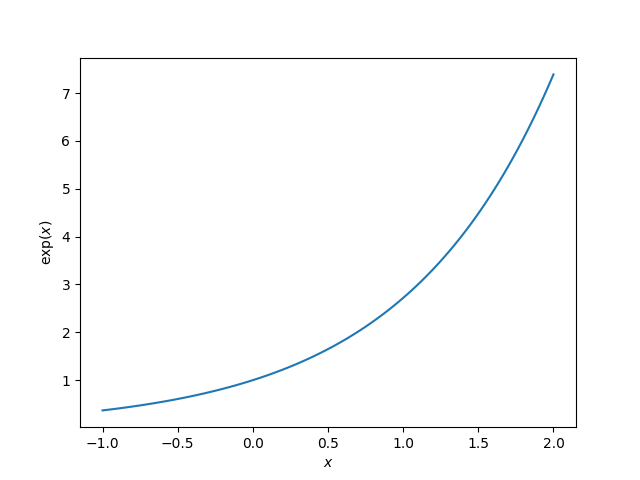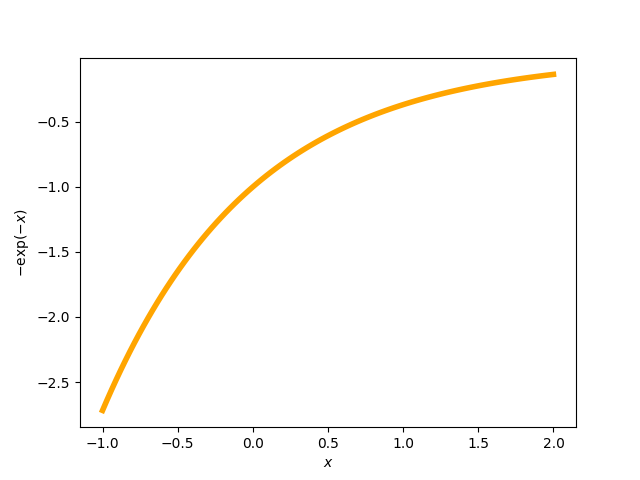# Choosing the thumbnail figure#

This example demonstrates how to choose the figure that is displayed as the thumbnail, if the example generates more than one figure. This is done by specifying the keyword-value pair `sphinx_gallery_thumbnail_number = <fig number>` as a comment somewhere below the docstring in the example file. In this example, we specify that we wish for the second figure to be the thumbnail.

••```# Code source: Óscar Nájera

import numpy as np
import matplotlib.pyplot as plt

def main():
"""Plot expoential functions."""
x = np.linspace(-1, 2, 100)
y = np.exp(x)

plt.figure()
plt.plot(x, y)
plt.xlabel("\$x\$")
plt.ylabel(r"\$\exp(x)\$")

# The next line sets the thumbnail for the second figure in the gallery
# (plot with negative exponential in orange)
# sphinx_gallery_thumbnail_number = 2
plt.figure()
plt.plot(x, -np.exp(-x), color="orange", linewidth=4)
plt.xlabel("\$x\$")
plt.ylabel(r"\$-\exp(-x)\$")
# To avoid matplotlib text output
plt.show()

if __name__ == "__main__":
main()
```

Total running time of the script: (0 minutes 1.161 seconds)

Estimated memory usage: 8 MB

Gallery generated by Sphinx-Gallery